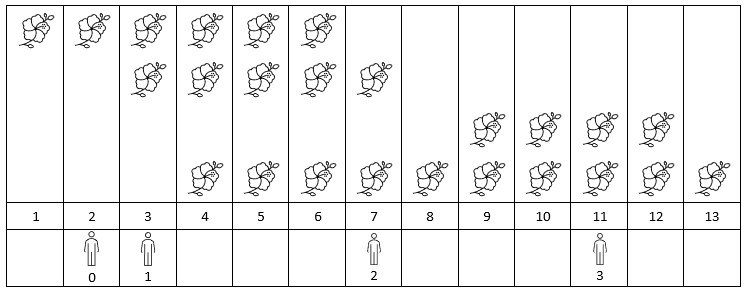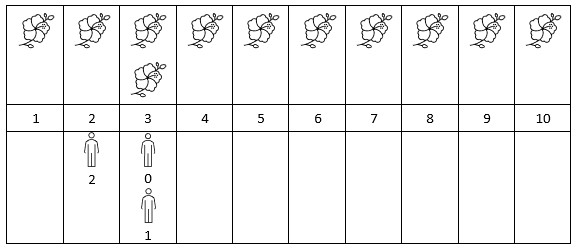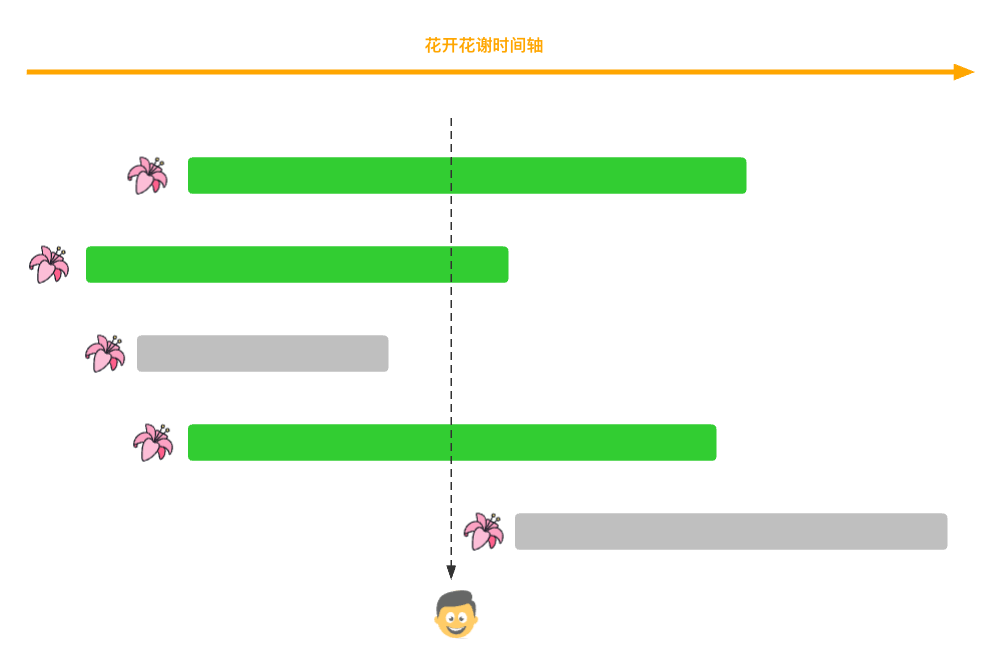#### 2251. 花期内花的数目(看官方题解, 已解)

###### 来源: 每日一题 2023.09.28``````输入：flowers = [[1,6],[3,7],[9,12],[4,13]], people = [2,3,7,11]

````````````输入：flowers = [[1,10],[3,3]], people = [3,3,2]

``````

• 1 <= `flowers.length` <= 5 * \$10^4\$
• `flowers[i].length == 2`
• 1 <= \$start_i\$ <= \$end_i\$ <= \$10^9\$
• 1 <= `people.length` <= 5 * \$10^4\$
• 1 <= `people[i]` <= \$10^9\$
``````class Solution {
public int[] fullBloomFlowers(int[][] flowers, int[] persons) {

}
}
``````

#### 分析与题解

• HashMap缓存 结果: 思路错误, 时间超时, 运行失败 GG

当看这个这个题目的元素量级之后, 我就知道, 如果双层循环暴力方案一定会超时失败, 所以我就在想如何减少运行时间, 我首先想到的就是HashMap.

思路是这样, 当处于某一个人 `people[i]` 看花时间, 如果先前没有缓存, 我们就缓存当前时间所有开放花的个数, 这样下一次某一个的看花时间 与 `people[i]`看花时间相同, 我们直接从缓存中取出答案即可.

``````int time = people[i];
if(cache.get(time) != null) {
result[i] = cache.get(time);
continue;
}
int count = 0;
for(int j = 0; j < flowers.length; j++) {
if(flowers[j] <= time && flowers[j] >= time) {
count++;
}
}
cache.put(time, count);
result[i] = count;
``````

但是这样, 时间复杂度由于for的双层嵌套导致复杂度过高, 从而导致了超时失败.

整体逻辑代码如下所示.

``````class Solution {
public int[] fullBloomFlowers(int[][] flowers, int[] people) {
// 暴力法一般会导致超时
// 解决方案: 时间复杂度降维

// 当某个时间点来人之后, 我们就把当前时间节点的开花数目添加缓存中
Map<Integer, Integer> cache = new HashMap<Integer, Integer>();
int[] result = new int[people.length];
for(int i = 0; i < people.length; i++) {
int time = people[i];
if(cache.get(time) != null) {
result[i] = cache.get(time);
continue;
}
int count = 0;
for(int j = 0; j < flowers.length; j++) {
if(flowers[j] <= time && flowers[j] >= time) {
count++;
}
}
cache.put(time, count);
result[i] = count;
}
return result;
}
}
``````

复杂度分析:

• 时间复杂度: O(nm), `n``people` 的数组长度成线性关系, `m``flowers` 的长度成线性关系.
• 空间复杂度: O(n), `n``people` 的数组长度成线性关系.

结果如下所示.

结果: 思路错误, 时间超时, 运行失败 GG

• 官方解法:二分法 结果: 成功

对于一个 `people[i]` 来说, 我当时的考虑是它是一个时间点, 而每一朵花的花期是一个时间区间, 当时在想如何降维处理, 就是对于 `people[i]` 如何不通过遍历花期数组, 找到符合要求的花. 也就是把时间复杂度将至 `O(n)` 而不是 `O(nm)`.

这样显然是不可行的. 那么官方是怎么做的呢? 官方有两种方式, 一种是查分, 一种是二分法, 这里我现在使用二分法.

首先, 我们看一下二分法的实现思路.

对于一个 `people[i]` 来说, 如果我们知道, 有 `x` 朵在 `people[i]` 时间点之前开花, 有 `y` 朵在 `people[i]` 时间点之前谢花. 我们就知道在 `people[i]` 时间点还未花谢的有 `x - y` 朵花, 如图所示.

首先, 谢花时间点一定在开花之后; 在某一个 `people[i]` 时间点之前就花谢, 那一定看不到这个花(`图中第三朵花`); 在某一个 `people[i]` 时间点之后就花开, 那也一定看不到这个花(`图中第五朵花`);

所以这个人能看到 `4 - 1 = 3` 朵花.如果我们没有排序, 对于每一个 `people[i]` 时间节点, 我们都需要遍历整个 `flowers` 数组, 时间复杂度是不会下降的.

所以对于这个题目来说, 我们首先对`flowers` 数组进行分组, 然后进行排序.

``````int[] start = new int[flowers.length];
int[] end = new int[flowers.length];
// 查找花期
for(int i = 0; i < flowers.length; i++) {
start[i] = flowers[i];
end[i] = flowers[i];
}
Arrays.sort(start);
Arrays.sort(end);
``````

然后利用二分法思想, 去查找个数.

``````// 优化操作: 二分法思想
int[] result = new int[persons.length];
for(int i = 0; i < persons.length; i++) {
int startCount = flowersCount(start, persons[i] + 1);
int endCount = flowersCount(end, persons[i]);
result[i] = startCount - endCount;
}
``````

我们看一下官方提供的二分法思路

``````public int flowersCount(int[] array, int target) {
int count = array.length;
int left = 0;
int right = array.length - 1;
while(left <= right) {
int mid = (left + right) >> 1;
if (array[mid] >= target) {
right = mid - 1;
count = mid;
} else {
left = mid + 1;
}
}
return count;
}
``````

上面的代码实际上有一个很有意思的优化点.

``````int mid = (left + right) >> 1;
``````

这个代码等同于如下.

``````int mid = (left + right) / 2;
``````

实际上就是利用位运算的右移运算进行了 `/2` 的操作, 但是他俩的效率差的不是一星半点. 我们看一下测试用例跑完的耗时.孰强孰弱就不过多解释了....

最后, 我们一起看一下整体的解题思路, 如下所示.

``````class Solution {
public int[] fullBloomFlowers(int[][] flowers, int[] persons) {
int[] start = new int[flowers.length];
int[] end = new int[flowers.length];
// 查找花期
for(int i = 0; i < flowers.length; i++) {
start[i] = flowers[i];
end[i] = flowers[i];
}
Arrays.sort(start);
Arrays.sort(end);
// 优化操作: 二分法思想
int[] result = new int[persons.length];
for(int i = 0; i < persons.length; i++) {
int startCount = flowersCount(start, persons[i] + 1);
int endCount = flowersCount(end, persons[i]);
result[i] = startCount - endCount;
}
return result;
}

public int flowersCount(int[] array, int target) {
int count = array.length;
int left = 0;
int right = array.length - 1;
while(left <= right) {
int mid = (left + right) >> 1;
if (array[mid] >= target) {
right = mid - 1;
count = mid;
} else {
left = mid + 1;
}
}
return count;
}
}
``````

复杂度分析:

• 时间复杂度: O((n+m)×logn) , `n``flowers` 的长度, `m``persons` 的长度, 排序需要的时间复杂度为 `O(nlogn)` , 对于 `persons[i]` 二分法的时间复杂度是 `O(logn)`, 一共有 `m` 个人, 所以总复杂度为 `O(mlogn)` , 最终整个题目的时间复杂度为 `O((n+m)×logn)`

• 空间复杂度: O(2n + logn), `start``end` 的开辟空间与 `flowers` 的长度成线性关系, 并且排序需要的空间复杂度是 `O(logn)`

结果如下所示.IT界无底坑洞栋主 欢迎加Q骚扰:676758285